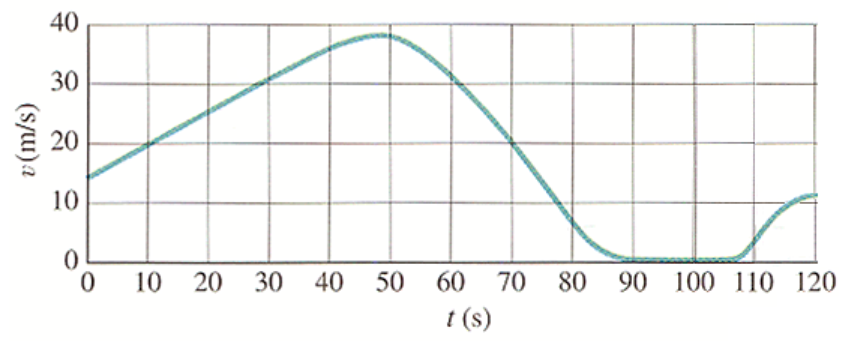# Problem: The figure below shows the velocity of a train as a function of time.(a) At what time was its velocity greatest?(b) During what periods, if any, was the velocity constant? (Enter these periods in the order in which they occur.)(c) During what periods, if any, was the acceleration constant? (Enter these periods in the order in which they occur.)(d) When was the magnitude of the acceleration greatest?

###### FREE Expert Solution

(a)

The velocity is greatest when the displacement from the time axis is highest.

The velocity is maximum at t = 48 s

(b)

The velocity is constant when the slope of the curve is horizontal.

82% (264 ratings)###### Problem Details

The figure below shows the velocity of a train as a function of time.

(a) At what time was its velocity greatest?
(b) During what periods, if any, was the velocity constant? (Enter these periods in the order in which they occur.)
(c) During what periods, if any, was the acceleration constant? (Enter these periods in the order in which they occur.)
(d) When was the magnitude of the acceleration greatest?